# Exponentiated Gradient

Exponentiated Gradient was introduced by Kivinen and Warmuth (1997) for the online linear regression problem. I can also be applied for prediction with expert advice, and in some sense can be classified to the category of exponential weights algorithms. In the simplest case, the algorithm predicts a sequence of numbers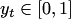using advice from a finite number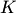of experts. The prediction space is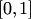. Each expert is initially assigned weight. The master's prediction is a weighted average of the experts' predictions. At each step the weights are updated by the exponential rule, which depends on the gradient of a loss function: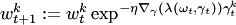, and then normalized. The intuituion behind this is that the weight is decreasing for the expert who adviced points in the direction of the largest increase of the loss function.

It is shown in Cesa-Bianchi (2006), that for the convex loss functions with a bounded gradient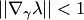and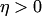the algorithm guarantees the inequality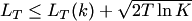for all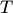and, where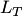is the loss suffered by master over the firsttrials, and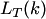is the loss suffered by theth expert over the firsttrials. The best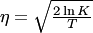.

For the online linear regression problem with bounded signal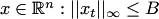, in Cesa-Bianchi (1999) you can find the proof that for the convex twice differentiable by the second argument loss function the Exponentiated Gradient algorithm can achieve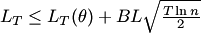,

where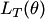is the loss of any linear function of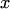, and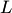is a bound for the derivative of the loss function: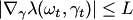. The best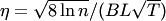.

### Bibliography

• Jyrki Kivinen and Manfred K. Warmuth: Exponentiated gradient versus gradient descent for linear predictors. Information and Computation 132(1):1-63, 1997.
• N. Cesa-Bianchi: Analysis of two gradient-based algorithms for on-line regression. Journal of Computer and System Sciences, 59(3):392-411, 1999.
• N. Cesa-Bianchi and G. Lugosi: Prediciton, Learning and Games. Cambridge University Press, 2006.